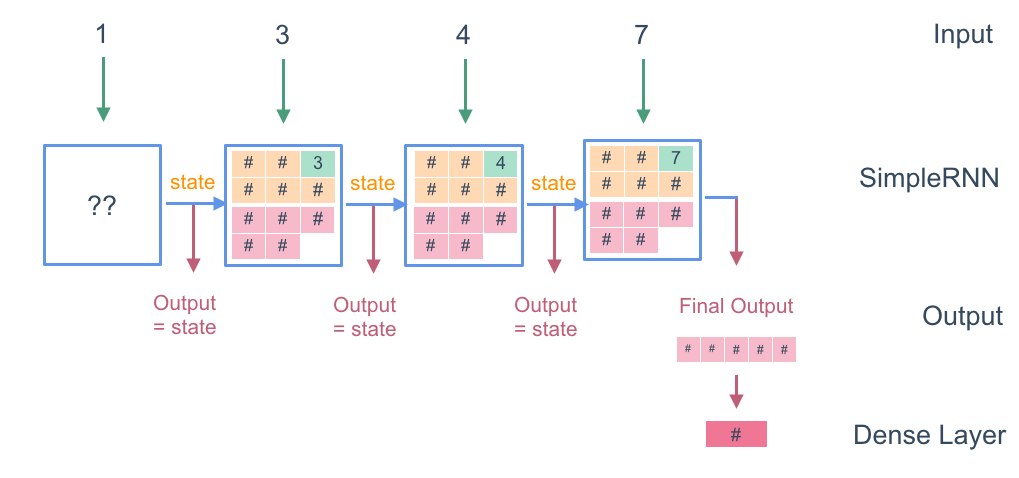# 6. Recurrent Neural Networks (RNNs)

In this tutorial we are going to look at Recurrent Neural Networks and time series data. In future videos, we are going to show how to take these RNNs and apply them to text data.

# Timeseries Data

First of all, what is time series data? In the real world, data changes over time. For instance, if we look at this famous dataset of airplane sales from 1949 - 1969 we can see that there is a general trend upwards, and a cyclical trend between years. In order to accurately predict the airline sales in 1960 for instance, we have to have some way of taking into account its time trend.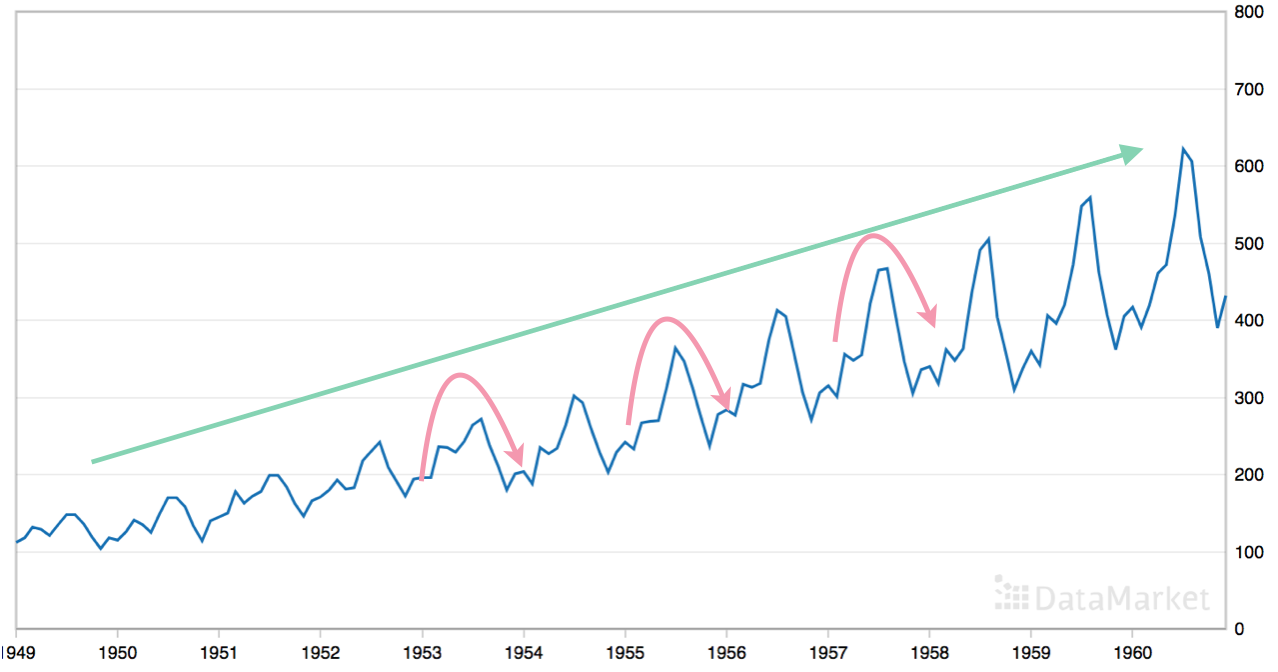Dataset of airline sales from 1949 - 1969

So how can we convert this data over time into the machine learning API (fixed length of numbers in and fixed length of numbers out)? We use a very simple and effective approach, known as the sliding window. We take a window of a fixed size, e.g. 10 elements, and put that in the first row of our training data. From these 10 elements, we want to output the 11th element, which we call the label. We keep sliding the window across, and eventually we will have a dataset that we can input into our perceptron, in the same way that we did in the very first tutorial in this series.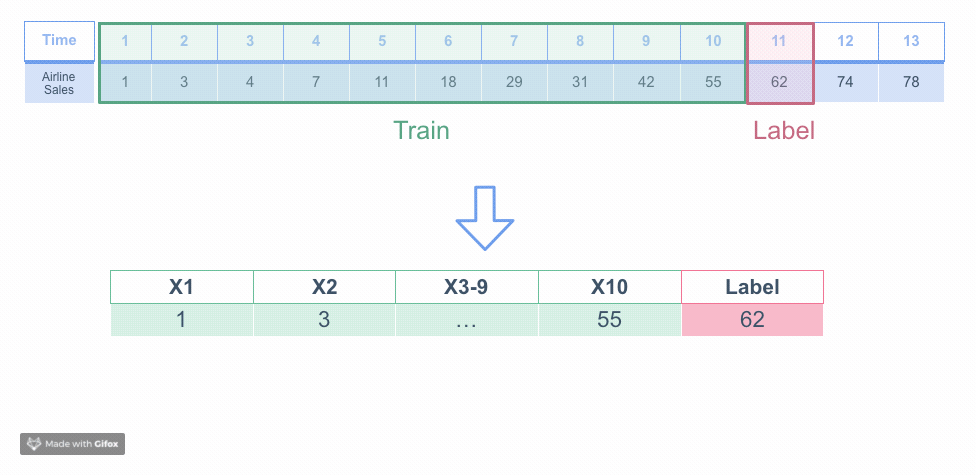Sliding window converts timeseries data into the Machine Learning API

# Code

Go into the timeseries directory in ml-class, and open up perceptron.py. This code sets up timeseries data and inputs it into a perceptron. Let’s walk through the code.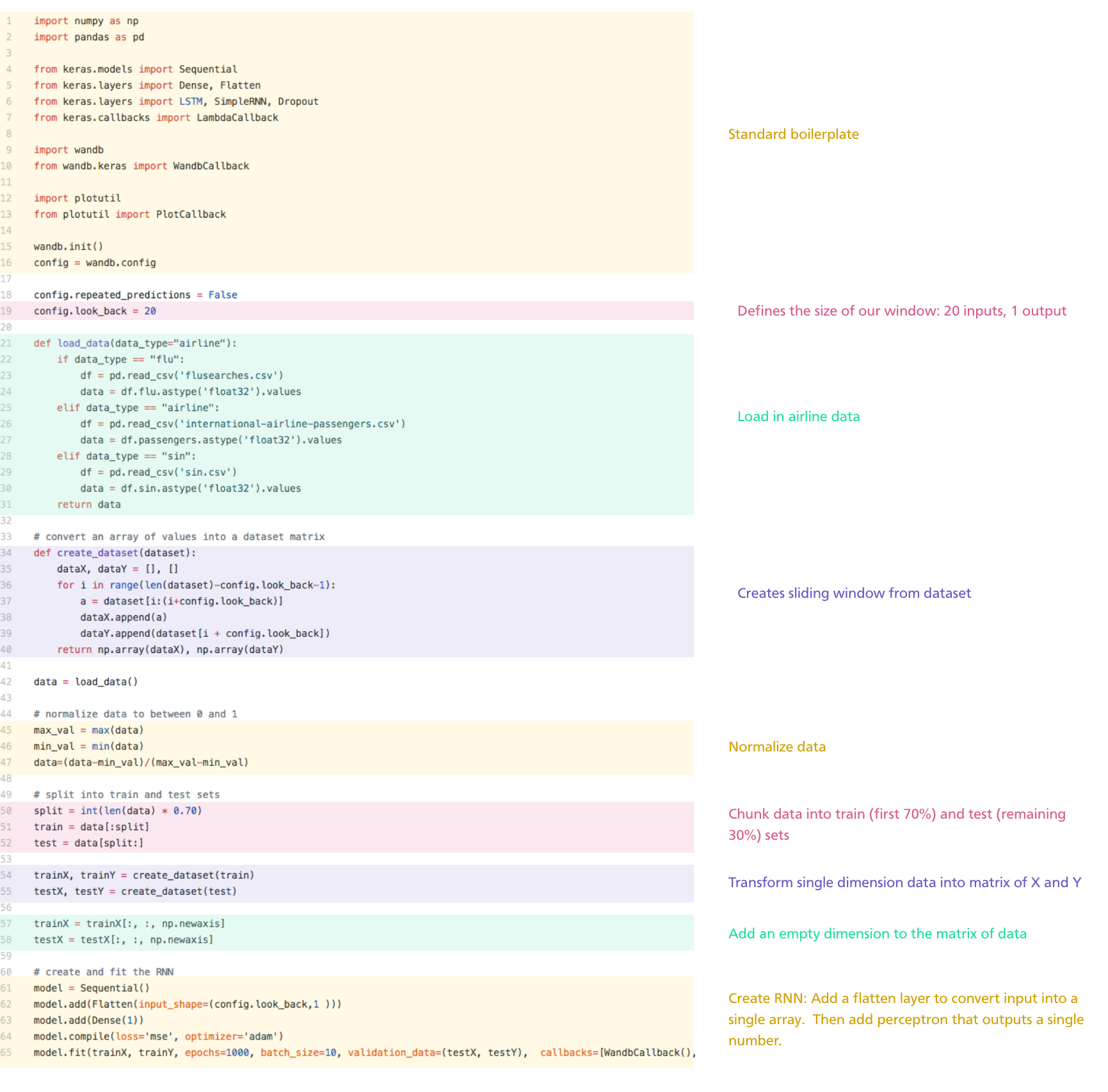Code in perceptron.py

# Recurrent Neural Networks

We have a pretty good accuracy from our simple perceptron, but we can improve it by using a Recurrent Neural Network (RNN). What’s better about an RNN than a perceptron? The difference is the causality of time. If you scramble the 20 inputs from the previous perceptron, it would have no effect on the prediction. But obviously from looking at the graph, we know that the order of those 20 events does matter. An RNN takes into account the order of the inputs, which becomes especially important on larger datasets.

Recurrent Neural Networks generally take in the same input as a dense neural network: they take in a vector of numbers over time and output a single number or a vector of numbers. The difference is that they keep track of a state, which they pass between themselves.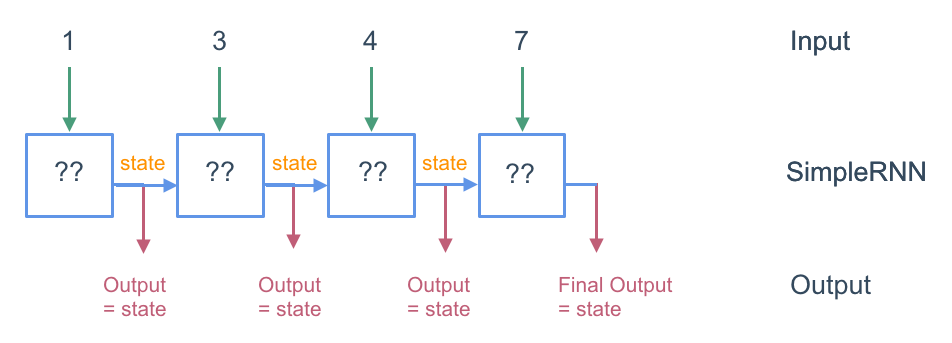Diagram of an RNN

So what happens inside the RNN? The RNN takes in a list of numbers (e.g. 1, 3, 4, 7), and at each step it passes along a state which it also outputs. In this case, the state is a single number. In this diagram of the second step, the network takes in the input 3 and it also takes in the state passed from the previous cell, 0.4. Using these two inputs, the network does a perceptron calculation - a weighted sum and a hyperbolic tangent activation function - and outputs a single number, 0.9. This output is then output by the cell and also passed to the next cell as a state. This same calculation is performed 10 times (or however long the window is) and then we take the final output to be the prediction of the label.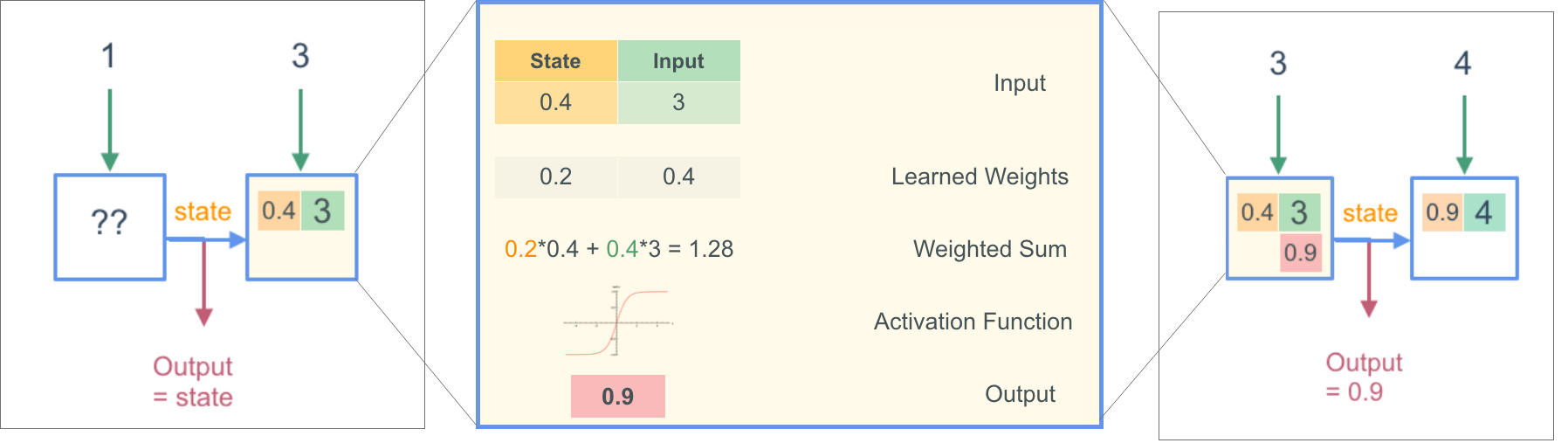Inside an RNN: Calculating the next state

What is a hyperbolic tangent activation function?
A hyperbolic tangent activation function acts like a sigmoid, but the values range from -1 to 1 instead of from 0 to 1.

We do the same tuning that we do with a perceptron, in which we perform backpropagation to calculate the best set of parameters to make the output what we want it to be.
Let’s see what this looks like in the code. Open up RNN.py. You will see that this is mostly the same, but on line 62 and 63 where we previously flattened the input and fed it into a dense layer, we now add a simple RNN layer:
//  line 62
The first 1 number means that the output and state at each time step is a single-dimensional number.

# Debugging the RNN

The first 1 number means that the output and state at each time step is a single-dimensional number.If we run rnn.py, we see that this model works a lot worse than the perceptron. Something isn’t working - let’s debug our model.

The reason our model is performing badly is because simply passing one number along from cell to cell is not enough for the model to learn the patterns in our data. We need to pass through more than one single number as a state. Let’s try and pass across a vector of 5 numbers instead. In the code change line 62 to:
//  line 62